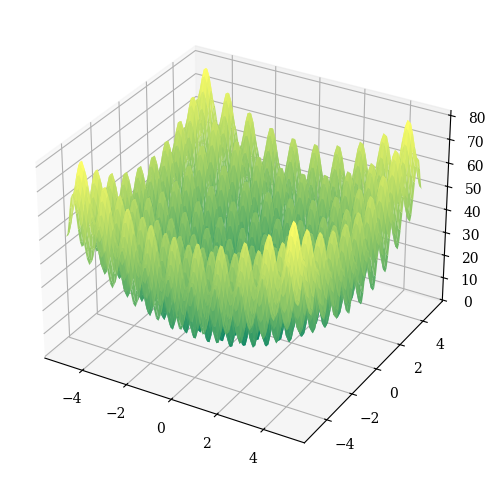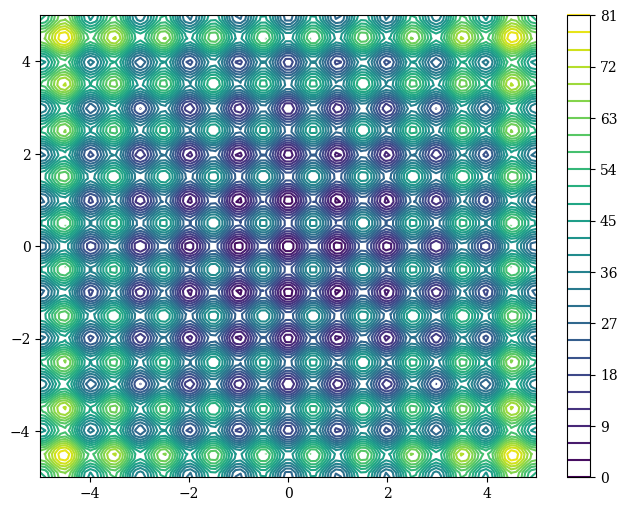Version: 0.6.0

# Rastrigin¶

The Rastrigin function has several local minima. It is highly multimodal, but locations of the minima are regularly distributed. It is shown in the plot above in its two-dimensional form.

Definition

\begin{align} \begin{split} f(x) &=& 10n + \sum\limits_{i=1}^n {[x_i^2 - 10 \cos{(2 \pi x_i)}]} \2mm] && -5.12 \leq x_i \leq 5.12 \quad i=1,\ldots,n \end{split} \end{align} Optimum \[f(x^*) = 0 \; \text{at} \; x^* = (0,\ldots,0)

Fitness Landscape

:

import numpy as np

from pymoo.problems import get_problem
from pymoo.visualization.fitness_landscape import FitnessLandscape

problem = get_problem("rastrigin", n_var=2)

FitnessLandscape(problem, angle=(45, 45), _type="surface").show()

:

<pymoo.visualization.fitness_landscape.FitnessLandscape at 0x7ff3d4c967f0>:

FitnessLandscape(problem, _type="contour", colorbar=True).show()

:

<pymoo.visualization.fitness_landscape.FitnessLandscape at 0x7ff3945ad400>